# GRE Problem Solving Question TypesWorried about what you need to know about problem solving on the GRE quant section? Never fear! We’ve compiled the basic GRE math question types here in one place so you can have a handy reference to return to as you study! First, we’ll go over the basic math concepts you’ll need, before taking a closer look at the breakdown of problem-solving question types.

## Math Concepts Tested in GRE Problem Solving

According to ETS (the test maker), here’s what GRE problem solving questions could test:

Pre-algebra: measuring, basic operations, statistics, probability, sequences, sets, ratios, proportions, percents, fractions, prime numbers, counting, permutations, combinations, and averages
Algebra: symbolism, exponents, mathematical expressions, percents, equations in one and two unknowns, word problems, quadratics, functions, factoring, and integers
Geometry: parallel lines, measure of angles, perimeter, area, circumference, triangles, quadrilaterals, circles, solids, coordinate geometry, and slope
Data Interpretation: charts, graphs, tables, and other pictorials

That sounds like a lot–and it is! But it’s important to remember two things. First of all, you’ll be able to master these through careful GRE quant review throughout your prep as a whole. Yes, it’s important to work on each concept in terms of problem solving questions specifically–but you’ll also be brushing up on them as you review other question types, too.

The second important thing to remember? An online calculator will be available for each test-taker.
“For individuals taking the test by computer, an online calculator will be provided for use in the quantitative sections.” The calculator features include addition, subtraction, multiplication, division, and a square root function.

## GRE Problem Solving Formats

These are (generally) four GRE Problem Solving formats. They are distinguished by their specific directions. Let’s examine these formats in a problem solving context.

### 1. Choose one answer choice.

Directions: This question has five answer choices. Select the best one of the answer choices given.

Example: If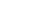and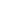are three distinct, positive integers each less than 10, what is the smallest possible value of the expression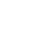1.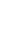2.3.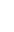4.5.The smallest possible value for the expression would be a fraction with the smallest possible numerator and the largest possible denominator. So, manipulate the positive integers less than 10 and envision the smallest possible fraction.

Let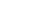Thus the denominator is 81. Then, letand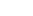. Thus,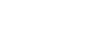.

### 2. Select all possible answer choices.

Directions: This question has five answer choices. Select all of the answer choices that are correct.

The correct answer to a question of this type could consist of one, two, three, four, or all five answer choices.

Example: If a farmer has 50 meters of fencing, which of the following areas can she enclose?

1.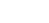2.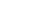3.4.5.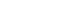A., B., C., and D. only are the correct answers.

A circle has the greatest area of any figure with a given perimeter (circumference). Accordingly, find the area of a circle with a circumference of 50m.

First find the radius: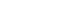then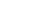, and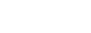.

Find the area:, then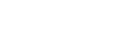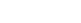. Anything less than this area can be enclosed. So, A., B., C., and D. are all correct.

### 3. Type-In answer (Numeric Entry).

Directions: This question does not have any answer choices. To answer this question enter an integer or a decimal in the answer space provided.

Example: What is the sixth term in the following sequence: 8, 4, 2, 1, 1/2, ….?

Click on the answer box, then type in a number. Backspace to erase.0.25 is the correct type-in answer.

This sequence is a geometric progression: each succeeding term equals the previous term multiplied by. So,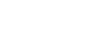. (Note that 0.25, .25, and .250 are all correct write-in responses.) Note that the test-maker expects you to respond with a decimal.

### 4. Select exactly three correct answer choices

Example: The weights of five packages are 1, 2, 5, 7, and 9 pounds. Which of the following CANNOT be the total weight, in pounds, of any combination of the packages?

1. 3
2. 4
3. 8
4. 11
5. 13
6. 20
7. 21
8. 25

B., F., and H. are the correct answers.

Plug In:

Evaluate each of the responses to determine whether it represents a possible total weight.

A. is possible: 1+2

B. is not possible

C. is not possible: 1+7

D. is possible: 2+9

E. is possible: 1+5+7

F. is not possible

G. is possible: 5+7+9

H. is not possible

Logical Deduction:

Start from the total weight of the packages: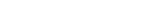and successively subtract weights of the smallest packages.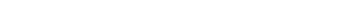etc.

• Two scored 30-minute Quantitative Reasoning Sections will typically appear on the GRE General Test.
• Several skills are tested on the Quantitative Reasoning sections.These include knowledge of mathematical principles, logical reasoning abilities, spatial skills, creativity, endurance, intuition, insight, patience, concentration, and the ability to perform under time constraints.
• Preparing for the exam experience involves a multifaceted approach. Plan to put in some serious study time.
• The GRE may include a pretest (experimental) math question set and will not be scored. There is no way to anticipate or identify a pretest question set. Don’t even think about it! Plan to do your best on every section.
• The quantitative questions drafted by the ETS test-makers may reflect as many as five difficulty gradients.
• Manage your time effectively throughout the math sections. A mix of approximately 20 math problems appears in two separate 30-minute sections. This means you’ll have about 1 3/4 minutes, on average, per question. By following our strategies, approaches, and shortcuts, you’ll learn to closely monitor your time so you’re able to consider each question carefully in the time allotted.

## Author

•Rachel is one of Magoosh’s Content Creators. She writes and updates content on our High School and GRE Blogs to ensure students are equipped with the best information during their test prep journey. As a test-prep instructor for more than five years in there different countries, Rachel has helped students around the world prepare for various standardized tests, including the SAT, ACT, TOEFL, GRE, and GMAT, and she is one of the authors of our Magoosh ACT Prep Book. Rachel has a Bachelor of Arts in Comparative Literature from Brown University, an MA in Cinematography from the Université de Paris VII, and a Ph.D. in Film Studies from University College London. For over a decade, Rachel has honed her craft as a fiction and memoir writer and public speaker. Her novel, THE BALLERINAS, is forthcoming in December 2021 from St. Martin's Press, while her memoir, GRADUATES IN WONDERLAND, co-written with Jessica Pan, was published in 2014 by Penguin Random House. Her work has appeared in over a dozen online and print publications, including Vanity Fair Hollywood. When she isn't strategically stringing words together at Magoosh, you can find Rachel riding horses or with her nose in a book. Join her on Twitter, Instagram, or Facebook!